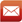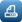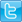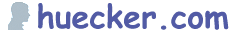GoTo: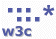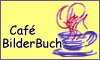Lesezeichen [ Info # QR-Code # Aphorismus ] Mo 14 Okt 2019 13:18:26

huecker.com # Grundlagen der Programmierung | Tcl Tutorial.
--

. Results of a command - Math 101 .

The Tcl command for doing math type operations is expr. The following discussion of the expr command is extracted and adapted from the expr man page.

Expr takes all of its arguments ("2 + 2" for example) and evaluates the result as a Tcl "expression" (rather than a normal command), and returns the value. The operators permitted in Tcl expressions include all the standard math functions, logical operators, bitwise operators, as well as math functions like rand(), sqrt(), cosh() and so on. Expressions almost always yield numeric results (integer or floating-point values).

Performance tip: enclosing the arguments to expr in curly braces will result in faster code. So do expr {\$i * 10} instead of simply expr \$i * 10.

OPERANDS
A Tcl expression consists of a combination of operands, operators, and parentheses. White space may be used between operands, operators and parentheses; it is ignored by the expression processor. Where possible, operands are interpreted as integer values. Integer values may be specified in decimal (the normal case), in octal (if the first character of the operand is 0), or in hexadecimal (if the first two characters of the operand are 0x).

Note that the octal and hexadecimal conversion takes place differently in the expr command than in the Tcl substitution phase. In the substitution phase, a \x32 would be converted to an ascii "2", while expr would covert 0x32 to a decimal 50.

If an operand does not have one of the integer formats given above, then it is treated as a floating-point number, if that is possible. Floating-point numbers may be specified in any of the ways accepted by an ANSI-compliant C compiler. For example, all of the following are valid floating-point numbers: 2.1, 3., 6e4, 7.91e+16. If no numeric interpretation is possible, then an operand is left as a string (and only a limited set of operators may be applied to it).

Operands may be specified in any of the following ways:

• As an numeric value, either integer or floating- point.
• As a Tcl variable, using standard \$ notation. The variable's value will be set as the operand.

OPERATORS
The valid operators are listed below, grouped in decreasing order of precedence:

- + ~ !
Unary minus, unary plus, bit-wise NOT, logical NOT. None of these operators may be applied to string operands, and bit-wise NOT may be applied only to integers.
* / %
Multiply, divide, remainder. None of these operators may be applied to string operands, and remainder may be applied only to integers. The remainder will always have the same sign as the divisor and an absolute value smaller than the divisor.
+ -
Add and subtract. Valid for any numeric operands.
<< >>
Left and right shift. Valid for integer operands only.
&
Bit-wise AND. Valid for integer operands only.
^
Bit-wise exclusive OR. Valid for integer operands only.
|
Bit-wise OR. Valid for integer operands only.
&&
Logical AND. Produces a 1 result if both operands are non-zero, 0 otherwise. Valid for numeric operands only (integers or floating-point).
||
Logical OR. Produces a 0 result if both operands are zero, 1 otherwise. Valid for numeric operands only (integers or floating-point).
x?y:z
If-then-else, as in C. If x evaluates to non-zero, then the result is the value of y. Otherwise the result is the value of z. The x operand must have a numeric value.

MATH FUNCTIONS
Tcl supports the following mathematical functions in expressions:

 acos cos hypot sinh asin cosh log sqrt atan exp log10 tan atan2 floor pow tanh ceil fmod sin

TYPE CONVERSIONS
Tcl supports the following functions to convert from one representation of a number to another: abs double int round.

--

. Example .

set X 100;
set Y 256;
set Z [expr "\$Y + \$X"]
set Z_LABEL "\$Y plus \$X is "

puts "\$Z_LABEL \$Z"
puts "The square root of \$Y is [expr sqrt(\$Y)]\n"

puts "Because of the precedence rules \"5 + -3 * 4\" is: [expr -3 * 4 + 5]"
puts "Because of the parentheses      \"(5 + -3) * 4\" is: [expr (5 + -3) * 4]"

puts "\n................. more examples of differences between  \" and \{"
puts {\$Z_LABEL [expr \$Y + \$X]}
puts "\$Z_LABEL {[expr \$Y + \$X]}"
puts "The command to add two numbers is: \[expr \\$a + \\$b]"

 --[ Home | Top ] [ . Previous | Index | Next . ]
Der Inhalt dieser Seite wurde am 24.05.2016 um 12.17 Uhr aktualisiert.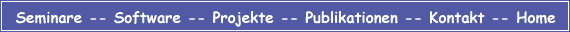huecker dot com * Germany | Datenschutz © 1999, 2019 Franz-Josef Hücker. All Rights Reserved.